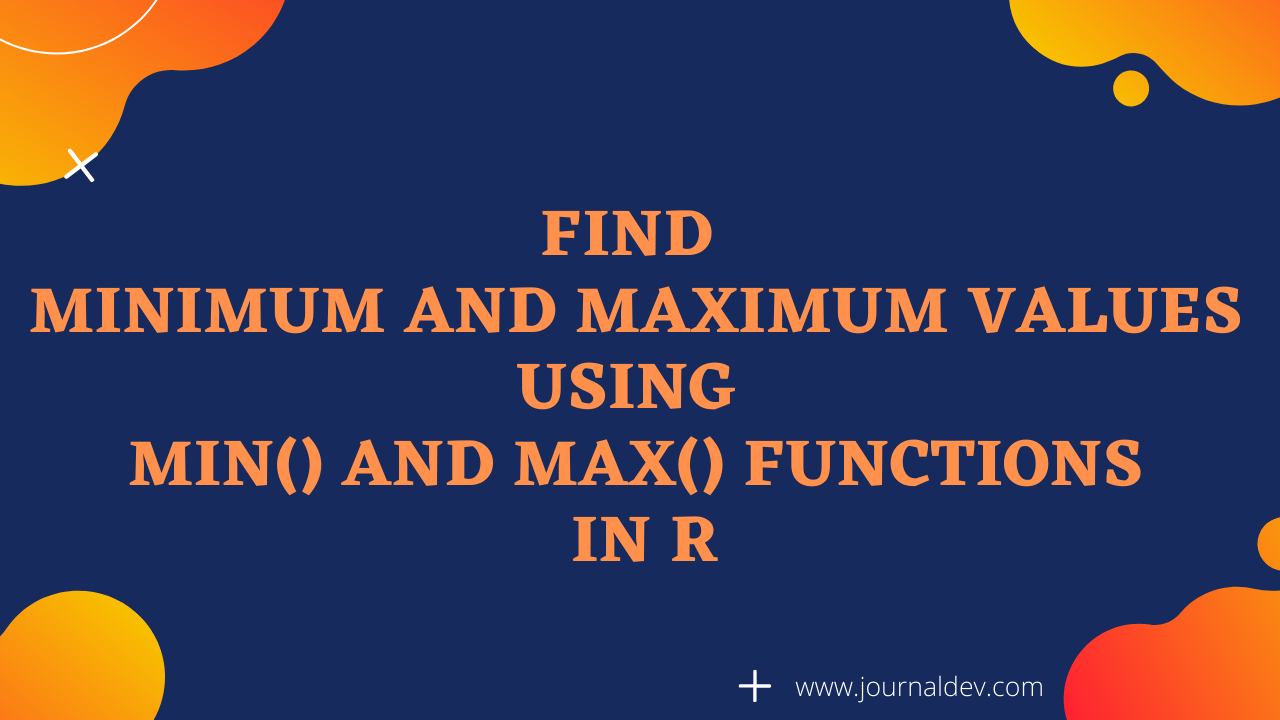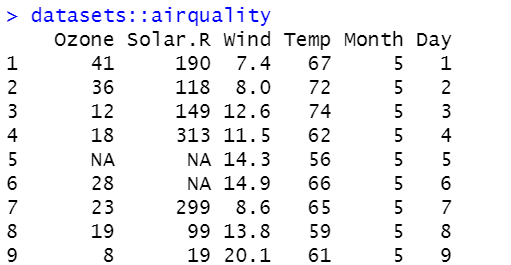# How to Use min() and max() in R

Published on August 3, 2022By Prajwal CNWhile we believe that this content benefits our community, we have not yet thoroughly reviewed it. If you have any suggestions for improvements, please let us know by clicking the “report an issue“ button at the bottom of the tutorial.

Finding min and max values is pretty much simple with the functions min() and max() in R.

You know that we have functions like mean, median, sd, and mode to calculate the average, middle, and dispersion of values respectively. But did you ever thought of a function which gives you min and max values in a vector or a data frame?

If so, congratulations, you have got functions named min() and max() which returns the minimum and maximum values respectively.

Sounds interesting right? Let’s see how it works!

The syntax of the min() function is given below.

``````min(x, na.rm = FALSE)
``````
• x = vector or a data frame.
• na.rm = remove NA values, if it mentioned False it considers NA or if it mentioned True it removes NA from the vector or a data frame.

The syntax of the max() function is given below.

``````max(x, na.rm = FALSE)
``````
• x = vector or a data frame.
• na.rm = remove NA values, if it mentioned False it considers NA or if it mentioned True it removes NA from the vector or a data frame.

## Max() function in R

In this section, we are going to find the max values present in the vector. For this, we first create a vector and then apply the max() function, which returns the max value in the vector.

``````#creates a vector
vector<-c(45.6,78.8,65.0,78.9,456.7,345.89,87.6,988.3)

#returns the max values present in the vector
max(vector)
``````

Output= 988.3

## Min() function in R

Here, we are going to find the minimum value in a vector using function min(). You can create a vector and then apply min() to the vector which returns the minimum value as shown below.

``````#creates a vector
vector<-c(45.6,78.8,65.0,78.9,456.7,345.89,87.6,988.3)

#returns the minimum value present in the vector
min(vector)
``````

Output = 45.6

## Max() function in R with NA values

Sometimes in the data analysis, you may encounter the NA values in a data frame as well as a vector. Then you need to bypass the NA values in order to get the desired result.

The max function won’t return any values if it encounters the NA values in the process. Hence you have to remove NA values from the vector or a data frame to get the max value.

``````#creates a vector having NA values
df<- c(134,555,NA,567,876,543,NA,456)

#max function won't return any value because of the presence of NA.
max(df)

#results in NA instead of max value
Output = NA
``````

So to avoid this and get the max value we are using na.rm function to remove NA values from the vector. Now you can see that the max() function is returning the maximum value.

``````#max function with remove NA values is set as TRUE.
max(df, na.rm = T)
``````

Output = 876

## Min() function in R with NA values

Just like we applied the max function in the above section, here we are going to find the minimum value in a vector having NA values.

``````#creates a vector having NA values
df<- c(134,555,NA,567,876,543,NA,456)

#returns NA instead of minimum value
min(df)
``````

Output = NA

To overcome this, we are using na.rm function to remove NA values from the vector. Now you can that the min() function is returning the min value.

``````#creates a vector having NA values
df<- c(134,555,NA,567,876,543,NA,456)

#removes NA values and returns the minimum value in the vector
min(df, na.rm = T)
``````

Output = 134

## Min() and Max() functions in a character vector

Till now we have dealt with numerical minimum and maximum values. If I have to tell you something, I wish to say that you can also find the min and max values for a character vector as well. Yes, you heard it right!

Let’s see how it works!

In the case of character vectors, the min and max functions will consider alphabetical order and returns min and max values accordingly as shown below.

``````#creates a character vector with some names
character_vector<- c('John','Angelina','Smuts','Garena','Lucifer')

#returns the max value
max(character_vector)
``````

Output = “Smuts”

Similarly, we can find the minimum values in the character vector as well using min() function which is shown below.

``````#creates a character vector with some names
character_vector<- c('John','Angelina','Smuts','Garena','Lucifer')

#returns the minimum values in the vector
min(character_vector)
``````

Output = “Angelina”

## Min() and Max() functions in a data frame

Let’s find the minimum and maximum values of a data frame by importing it. The min and max values in a dataset will give a fair idea about the data distribution.

This is the air quality dataset that is available in R studio. Note that the dataset includes NA values. With the knowledge of removing NA values using na.rm function, let’s find the min and max values in the Ozone values.``````min(airquality\$Ozone, na.rm=T)
``````

Output = 1

``````max(airquality\$Ozone, na.rm = T)
``````

Output = 168

Let’s find the min and max values of the Temperature values in the airquality dataset.

`````` min(airquality\$Temp, na.rm = T)
``````

Output = 56

``````max(airquality\$Temp, na.rm = T)
``````

Output = 97

## Wrapping up

Well, in this tutorial we have focussed on finding the minimum and maximum values in a vector, data frame, and a character vector as well.

The min and max functions can be used in both numerical as well as character vectors. You can also remove the NA values using na.rm function to get better accuracy and desired results.

That’s all for now. I hope you will find more min and max values as shown in the above sections. Don’t forget to hit the comments section for any queries.

Happy learning!!!

More study: R documentation

Thanks for learning with the DigitalOcean Community. Check out our offerings for compute, storage, networking, and managed databases.Prajwal CN

author

#### Still looking for an answer?

Ask a questionSearch for more help

JournalDev
DigitalOcean EmployeeMarch 27, 2021

So min and max in order to compare character vector only compare the first alphabetic order and ignore the ascii value of uppercase and lowercase ??

- r_study_boomer

Click below to sign up and get \$200 of credit to try our products over 60 days!

### Popular Topics##### Hollie's Hub for Good

Working on improving health and education, reducing inequality, and spurring economic growth? We’d like to help.##### Become a contributor

You get paid; we donate to tech nonprofits.

##### Welcome to the developer cloud

DigitalOcean makes it simple to launch in the cloud and scale up as you grow – whether you’re running one virtual machine or ten thousand.© 2023 DigitalOcean, LLC.